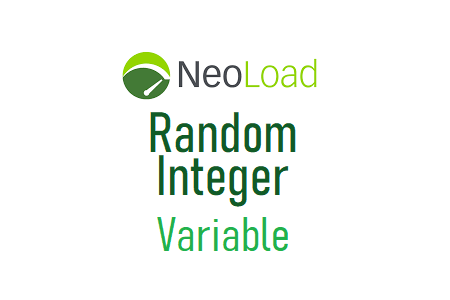# NeoLoad – Random Integer Variable‘Random Integer’ is a type of variable in NeoLoad to generate the integer number randomly. It is a simple type of number generator within the given range and help to simulate the random number logic in the performance test script. e.g. 10, 155, 26, 30 etc.

The very common use of ‘Random Integer’ is to input a number while filling a form. Like Age (Number Range 18 to 60), Weight (Number Range 40 to 120), Heigth (Number Range 50 to 250) etc.

### Inputs of ‘Random Integer’ type variable

1. Name: To provide the name of the variable to be used in the script. This is a mandatory input.
2. Description: To describe the use of the variable. This is an optional input.
3. Parameters: To configure the parameter setting:
1. Minimum value: Specify the minimum value of the number range
2. Maximum value: Specify the maximim value of the number range
3. Predictable randomization: Check-mark this option to make the same sequence of the random number. This option helps to compare two identical tests.
4. Value change policy: To define the setting when the next row (data) will be picked
1. On each use (Default value)
2. On each request
3. On each page
4. On each iteration
5. For each Virtual User instance

### Example

Consider, a personal detail submission form where a person needs to fill in his/her details. The form contains the following fields:

1. Name
2. Age
3. Pin Code

The person name can be parameterized with the ‘Random String’ variable type, but to parametrize ‘Age’ and ‘Pin Code’; ‘Random Integer’ is the best option. Create two random integers and give the name ‘age’ and ‘pinCode’. The ‘Value change policy’ is set to ‘on each iteration’. Refer to the below screenshot for better understanding: